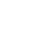# Henry's Law Constants

### Rolf Sander

Atmospheric Chemistry Division

Max-Planck Institute for Chemistry
Mainz, Germany

## Errata

#### Contact, Impressum, Acknowledgements

When referring to the compilation of Henry's Law Constants, please cite this publication:

R. Sander: Compilation of Henry's law constants (version 4.0) for water as solvent, Atmos. Chem. Phys., 15, 4399-4981 (2015), doi:10.5194/acp-15-4399-2015

# Henry's Law Constants → Organic species with phosphorus (P) → Phosphorus (C, H, O, N, Cl, Br, S, P) → tributyl phosphorotrithioite

 FORMULA: C12H27PS3 CAS RN: 150-50-5 STRUCTURE (FROM NIST):InChIKey: KLAPGAOQRZTCBI-UHFFFAOYSA-N

$Hscp$ $d ln Hs cp / d (1/T)$ Reference Type Notes
[mol/(m3Pa)] [K]
4.3×10−1 HSDB (2015) Q 38)
4.3×10−1 Zhang et al. (2010) Q 107) 108)
6.0×10−4 Zhang et al. (2010) Q 107) 109)
1.5×10−1 Zhang et al. (2010) Q 107) 110)
5.1×10−2 Zhang et al. (2010) Q 107) 111)

## References

• HSDB: Hazardous Substances Data Bank, TOXicology data NETwork (TOXNET), National Library of Medicine (US), URL http://toxnet.nlm.nih.gov/newtoxnet/hsdb.htm (2015).
• Zhang, X., Brown, T. N., Wania, F., Heimstad, E. S., & Goss, K.-U.: Assessment of chemical screening outcomes based on different partitioning property estimation methods, Environ. Int., 36, 514–520, doi:10.1016/J.ENVINT.2010.03.010 (2010).

## Type

Table entries are sorted according to reliability of the data, listing the most reliable type first: L) literature review, M) measured, V) VP/AS = vapor pressure/aqueous solubility, R) recalculation, T) thermodynamical calculation, X) original paper not available, C) citation, Q) QSPR, E) estimate, ?) unknown, W) wrong. See Section 3.1 of Sander (2015) for further details.

## Notes

 38) Calculated based on the method by Meylan and Howard (1991). 107) Data taken from the supplement. 108) Calculated using the EPI Suite (v4.0) method. 109) Calculated using the SPARC (v4.2) method. 110) Calculated using the COSMOtherm (v2.1) method. 111) Calculated using the ABSOLV (ADMEBoxes v4.1) method.

The numbers of the notes are the same as in Sander (2015). References cited in the notes can be found here.

# * * *

## Convert Henry's Law Constants

Convert: mol/(m3*Pa) (Hscp) M/atm (Hscp) 1/atm (Hsxp) mol/(kg*Pa) (Hsbp) mol/(kg*atm) (Hsbp) (dimensionless aq/g) (Hscc) m3*Pa/mol (Hvpc) m3*atm/mol (Hvpc) atm (Hvpx) (dimensionless g/aq) (Hvcc) (dimensionless aq/g) (alpha)